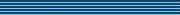| home      | people     | research     | publications    | seminars    | events     | contactCommunication Networks   | Systems Biology   | Hybrid Systems    | Machine Learning   | Dynamics & Interaction### Schools Mathematics Grand Challenge

Week one's Puzzles

Problem 1:

The solution was:

Let's calculate the total amount of money Mary would get from each bank in turn.

```Bank 1: 1000 x (1.3) = 1300
Bank 2: 1000 x (1.15) x (1.15) = 1322.50
Bank 3: 1000 x (1.1) x (1.1) x (1.1) = 1331
Bank 4: 1000 x (1.075) x (1.075) x (1.075) x (1.075) = 1335.47
Bank 5: 1000 x (1.06) x (1.06) x (1.06) x (1.06) x (1.06) = 1338.23
Bank 6: 1000 x (1.05) x (1.05) x (1.05) x (1.05) x (1.05) x (1.05) = 1340.09
```

So the answer is 6.

Problem 2:

The solution was:

The 4 conditions mean that the length of a week under the new scheme must be a whole number other than 1 and 365 that divides evenly into 365. The prime factors of 365 are 5 and 73. No other positive whole numbers (apart from 1 and 365) will divide evenly into 365. Thus we have only 2 options for the length of a week - 5 days or 73 days so the answer is 2.

While a 73 day week would be painful, the two week break at Christmas might compensate!

Problem 3:

The solution was:

The person who speaks first tells the truth and is wearing a blue hat. This tells us that the first speaker cannot be John.

Sheila then replies to the first speaker, so the first speaker is not Sheila either. Thus the first speaker must be Mark. Thus Mark is wearing a blue hat and the answer is 2.